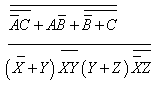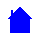ASSIGNMENT #4

```
1. Perform the following subtractions using 2's complement addition and
an 8-bit number size

82- 59
73 - 117

2. Perdorm the following subtractions using 1's complement addition and
an 8-bit number size

25 - 113
125 - 43

3. Simplify the following expressions using Demorgan's theorem, as required```ELET1210 Homepage